This work was in part supported by the National Science Foundation-Department of Energy (DOE) partnership for plasma science and engineering (grant DE-SC0016248) and by the DOE-National Nuclear Security Administration (grant DE-NA0003842)

First-Principles Equation of State (FPEOS) Database for Warm Dense Matter Computation

 Authors: B. Militzer, F. Gonzalez-Cataldo, S. Zhang, K. P. Driver, F. Soubiran

With the goal in mind of making WDM computations more reliable and efficient, we make available our EOS tables for 11 elements and 10 compounds as well as the C++ computer codes for their interpolation. Python code is provided to generate graphs of shock Hugoniot curve, isentropes, isobars, and isotherms for compounds and user-defined mixtures. We put together this first-principles equation of state (FPEOS) database for matter at extreme conditions by combining results from path integral Monte Carlo and density functional molecular dynamics simulations of the elements H, He, B, C, N, O, Ne, Na, Mg, Al and Si as well as the compounds LiF, B4C, BN, CH4, CH2, C2H3, CH, C2H, MgO, and MgSiO3. For all these materials, we provide the pressure and internal energy over a wide density-temperature range from ~0.5 to 50 g/cc and from ~104 to 109 K. This database encompasses the results from approximately 5000 different first-principles simulations. It allows one to compute isobars, adiabats, and shock Hugoniot curves in the regime of L and K shell ionization. Invoking the linear mixing approximation, one can study the properties of user-defined mixtures at high density and temperature.

Recommended citation: B. Militzer, F. Gonzalez-Cataldo, S. Zhang, K. P. Driver, F. Soubiran, "First-Principles Equation of State Database for Warm Dense Matter Computation", Physical Review E 103 (2021) 013203.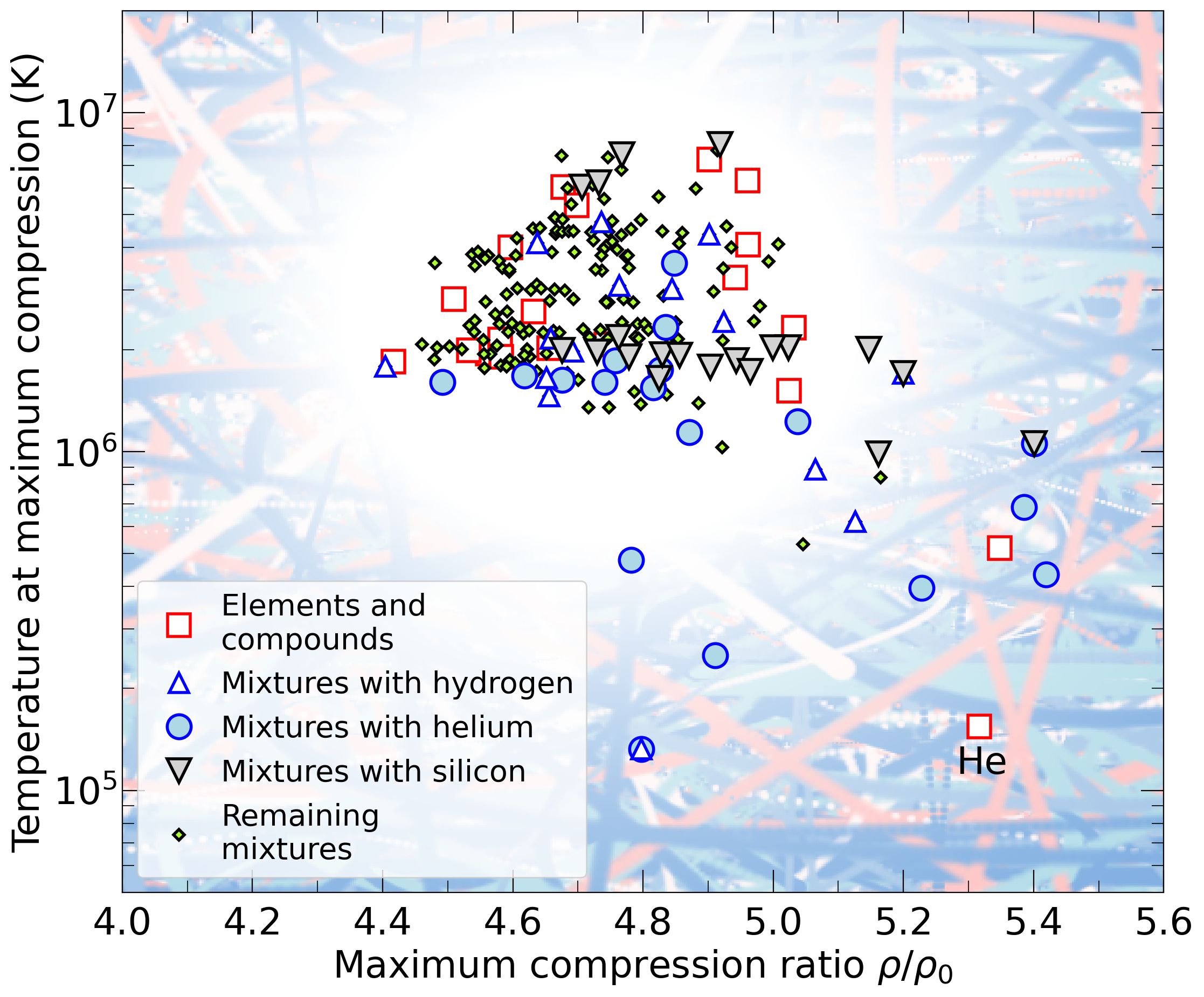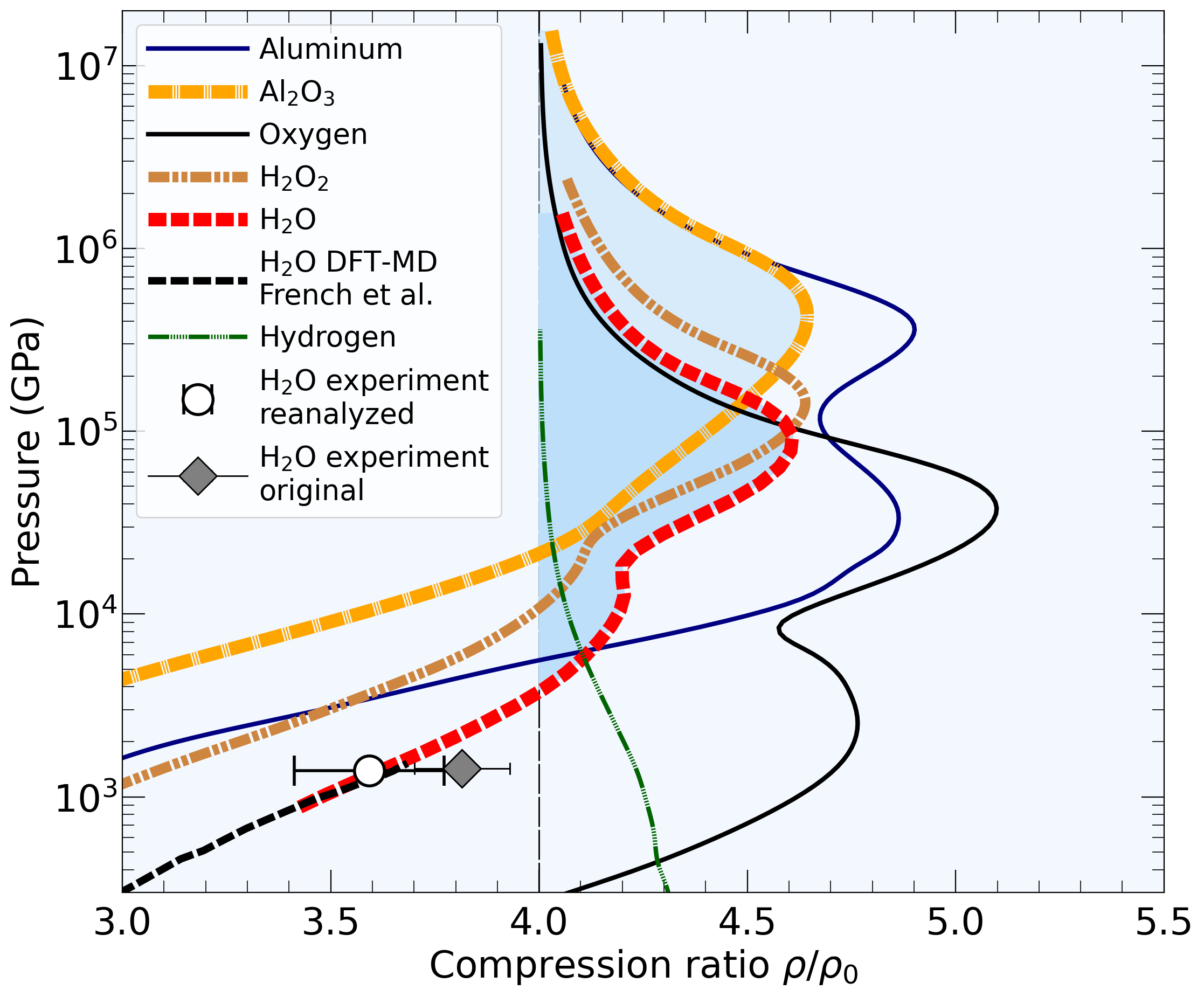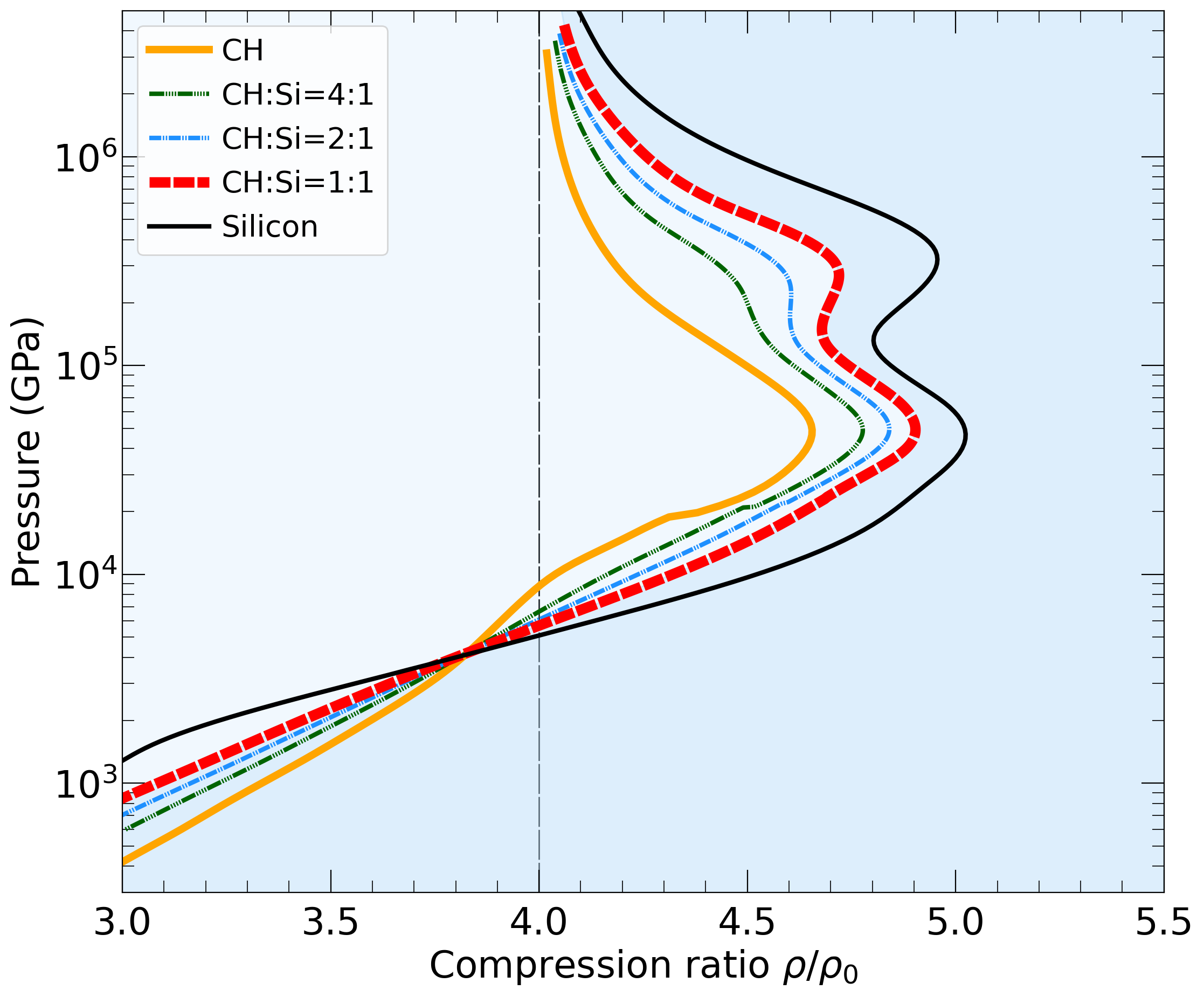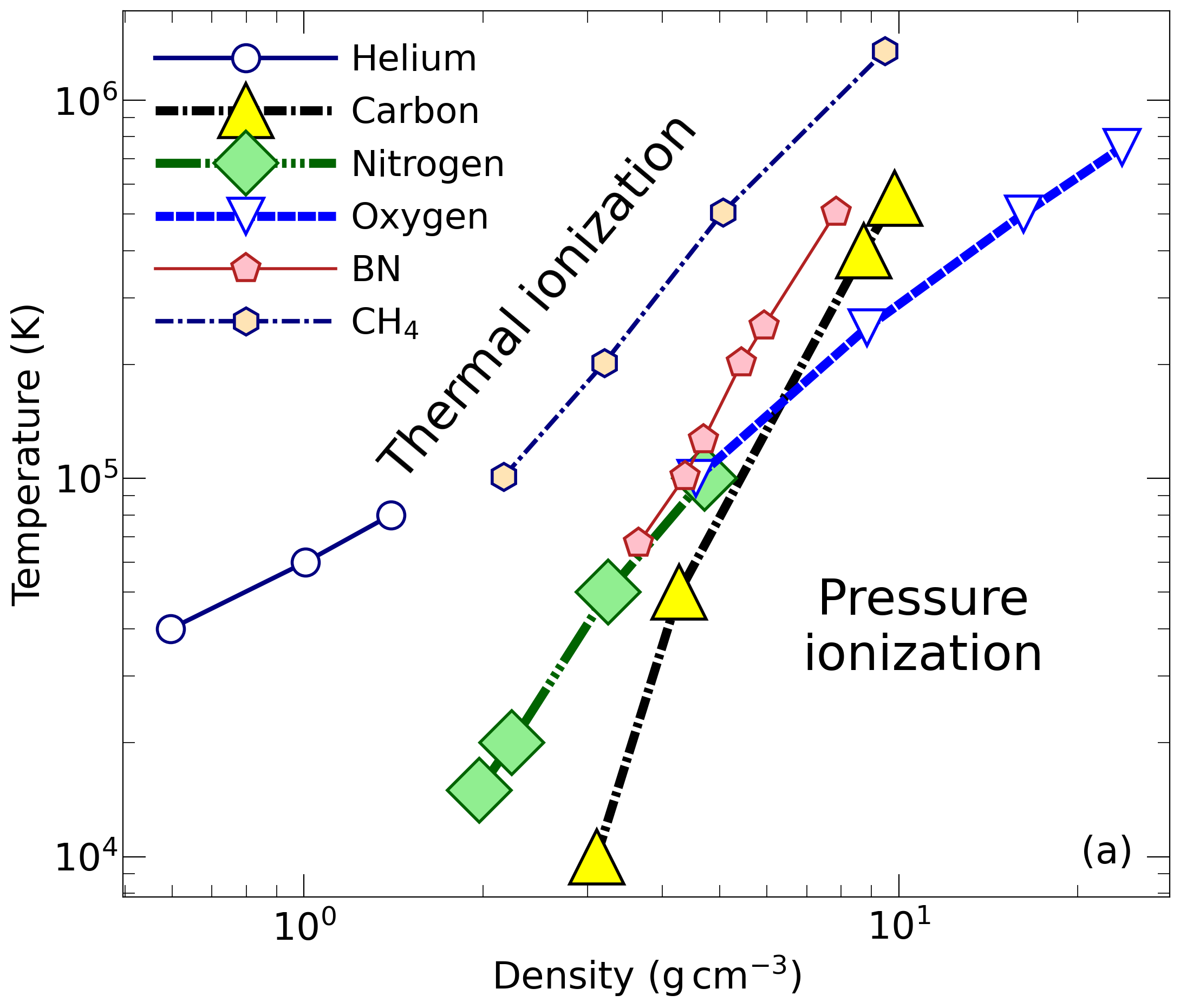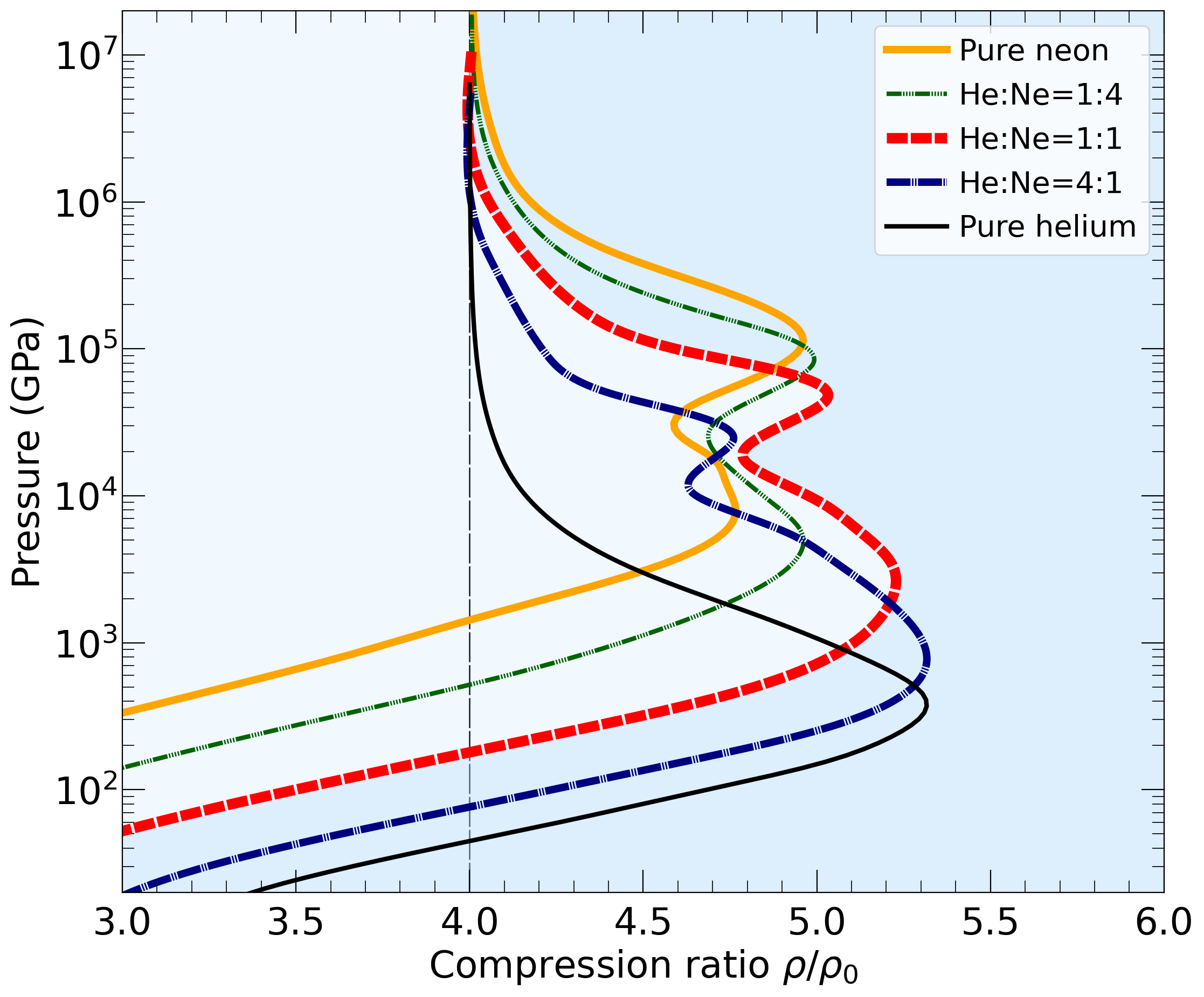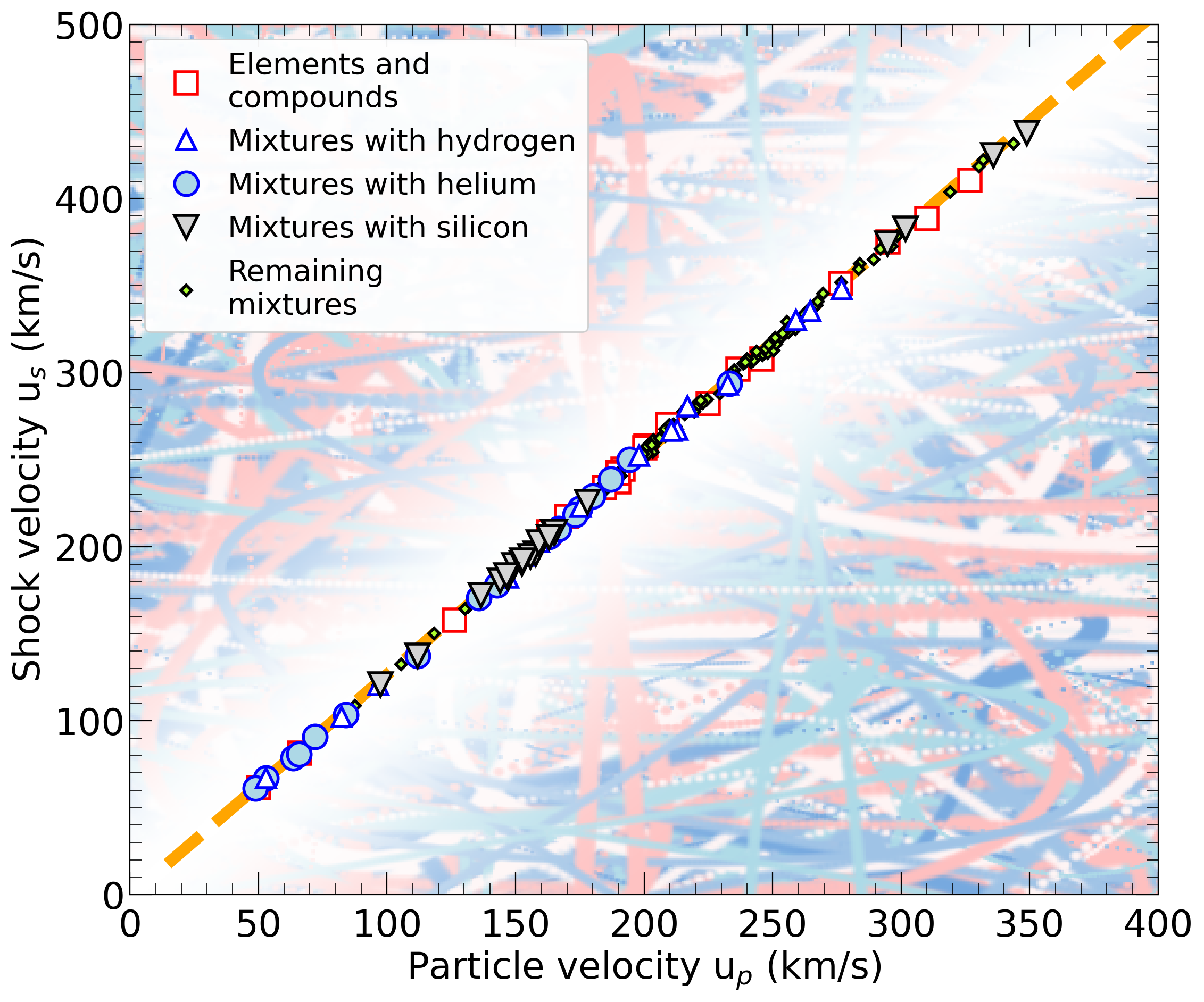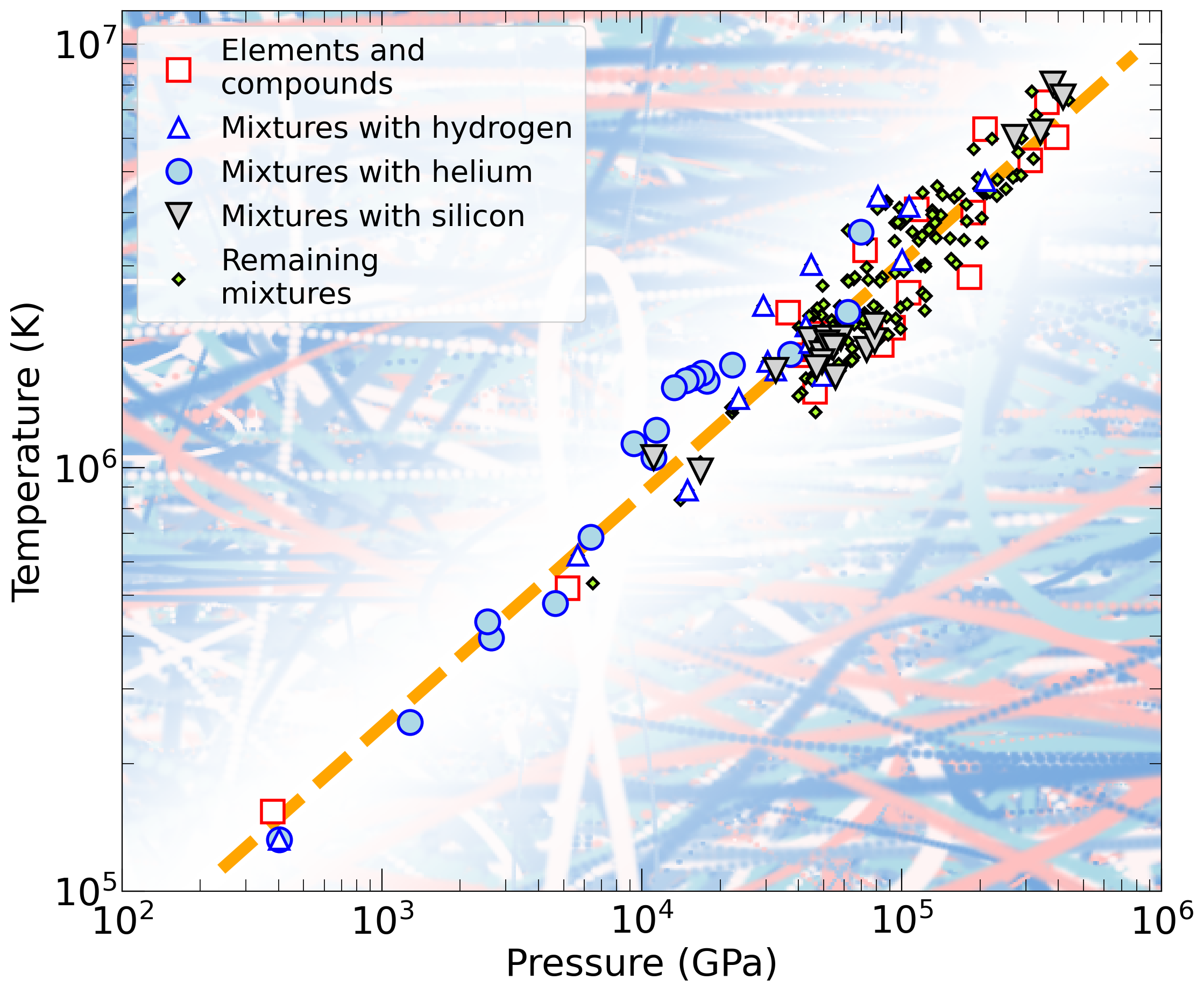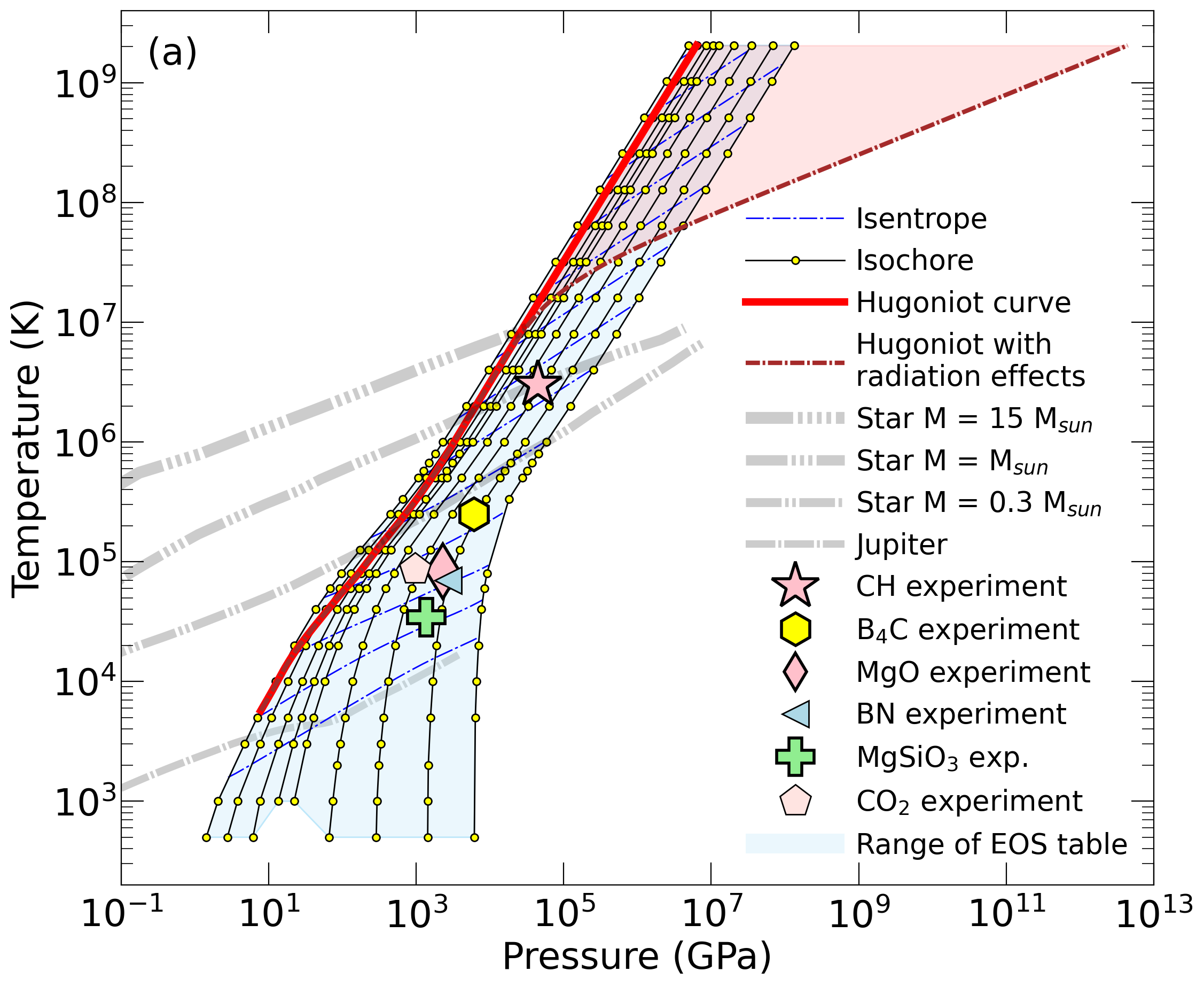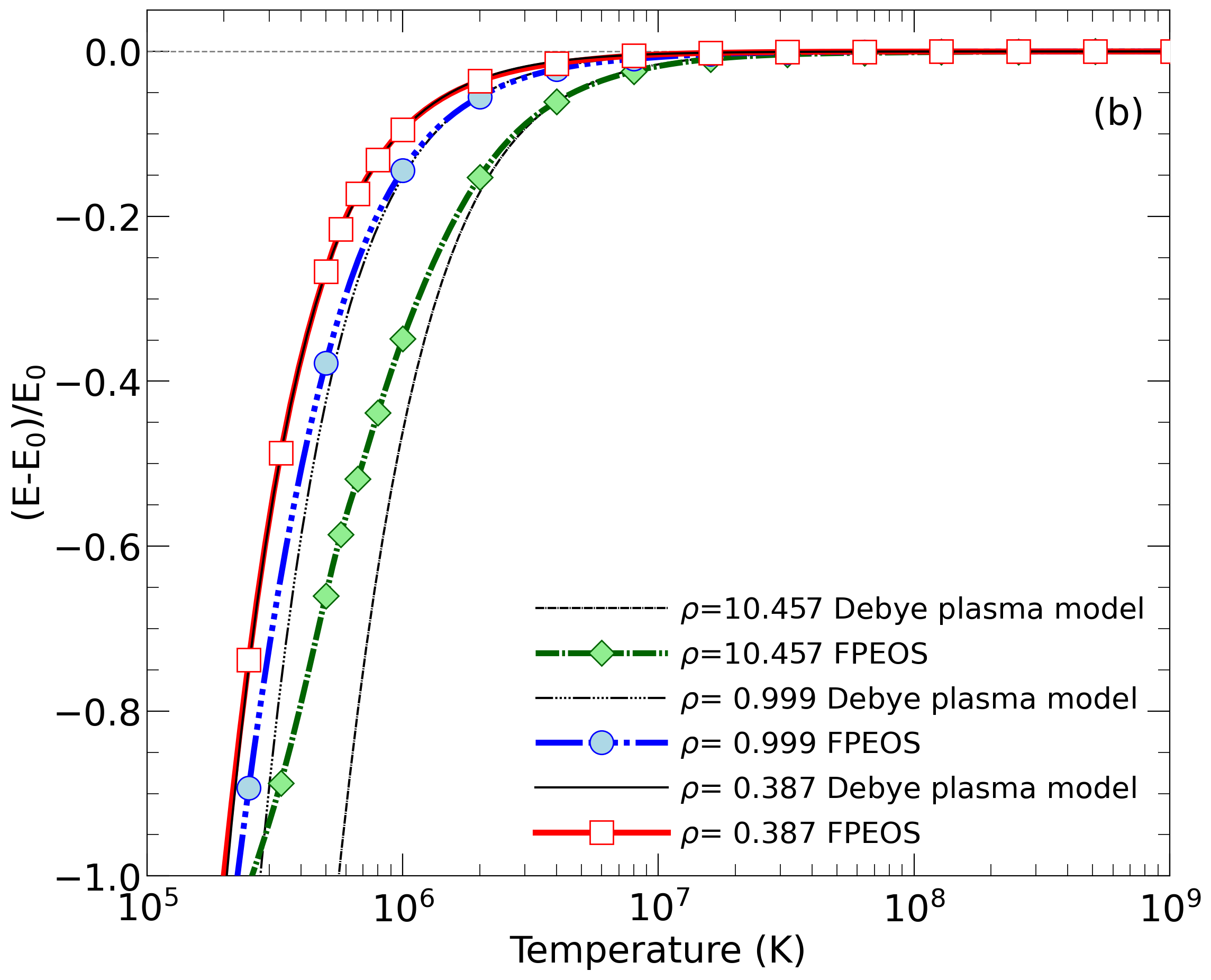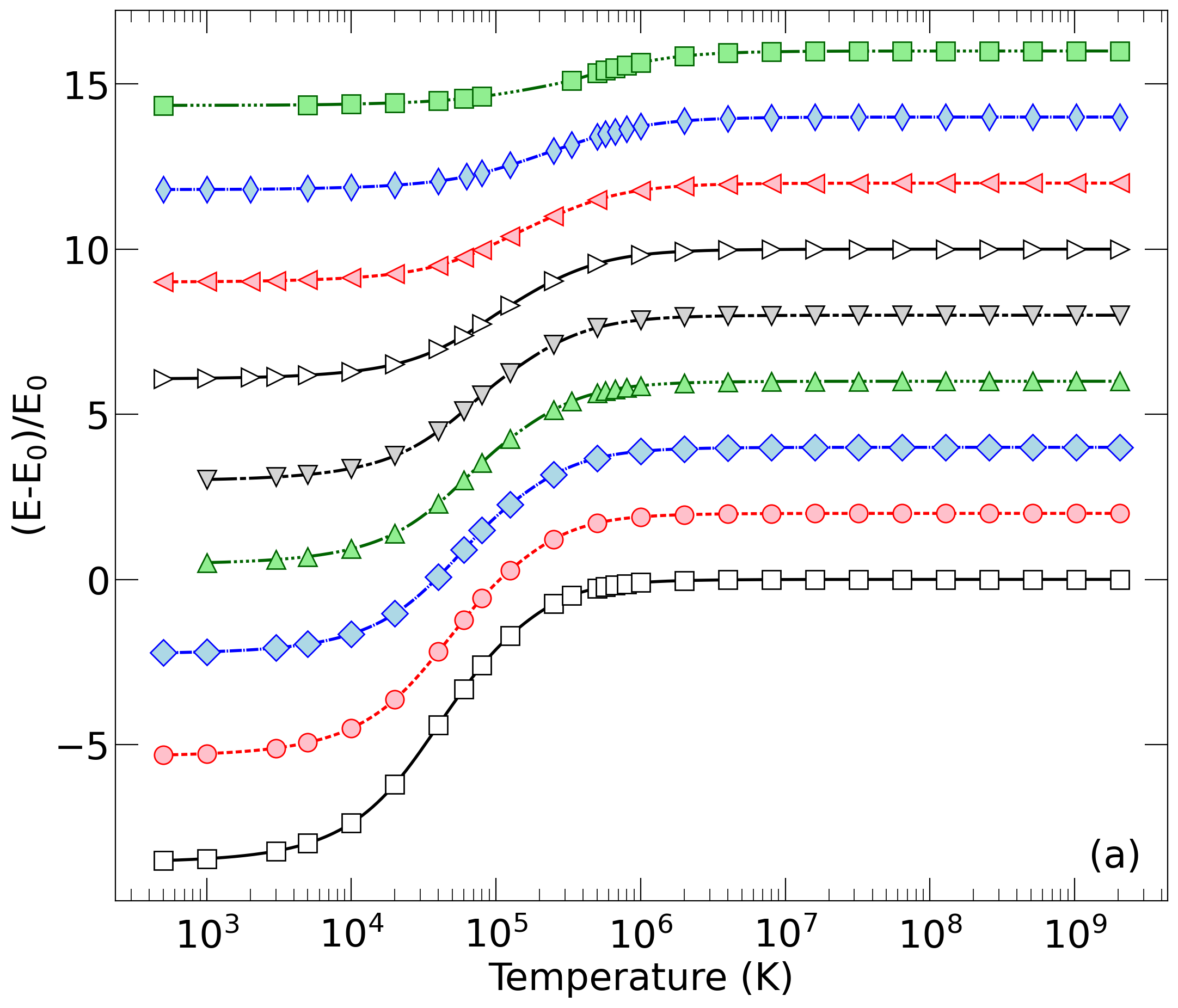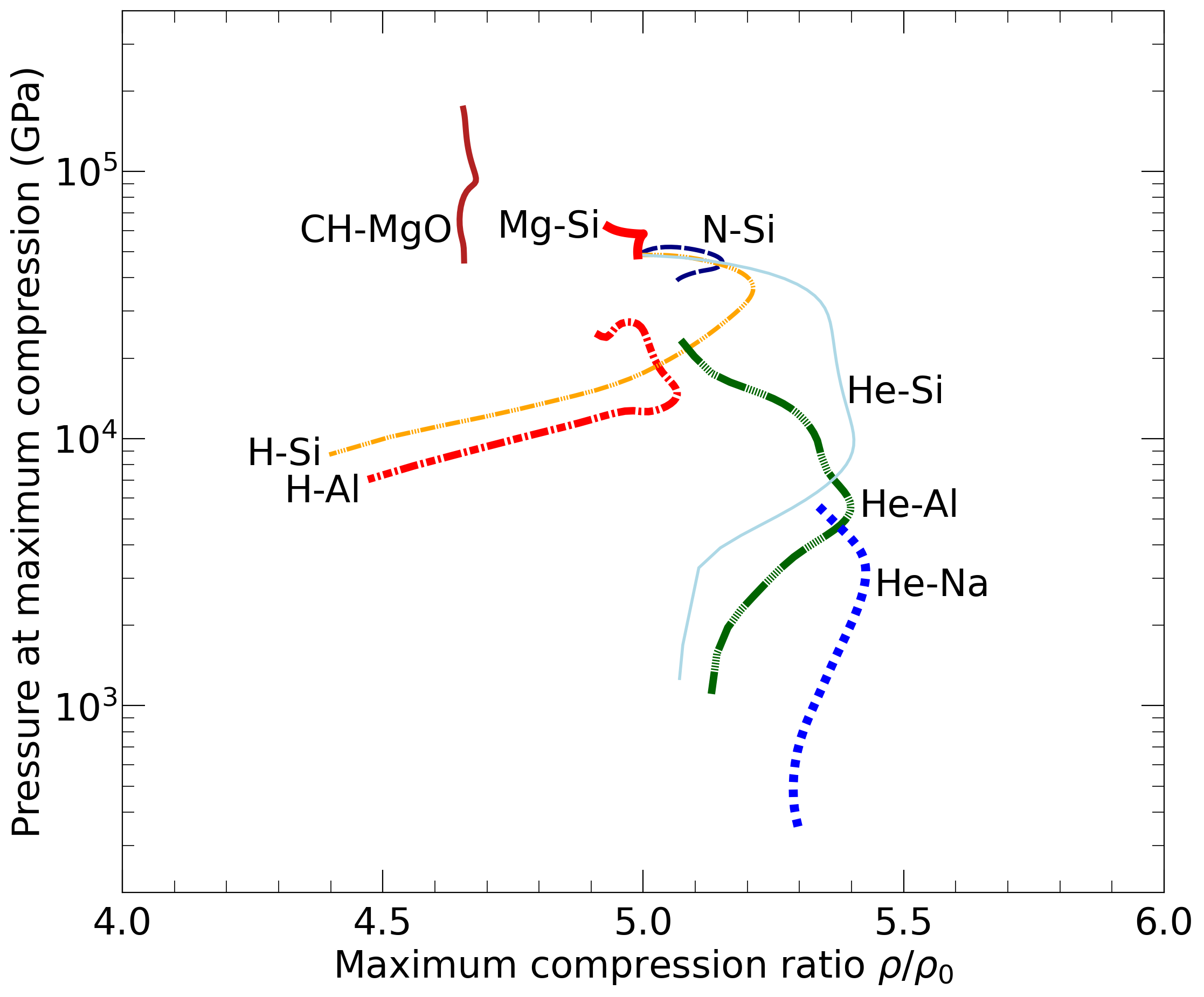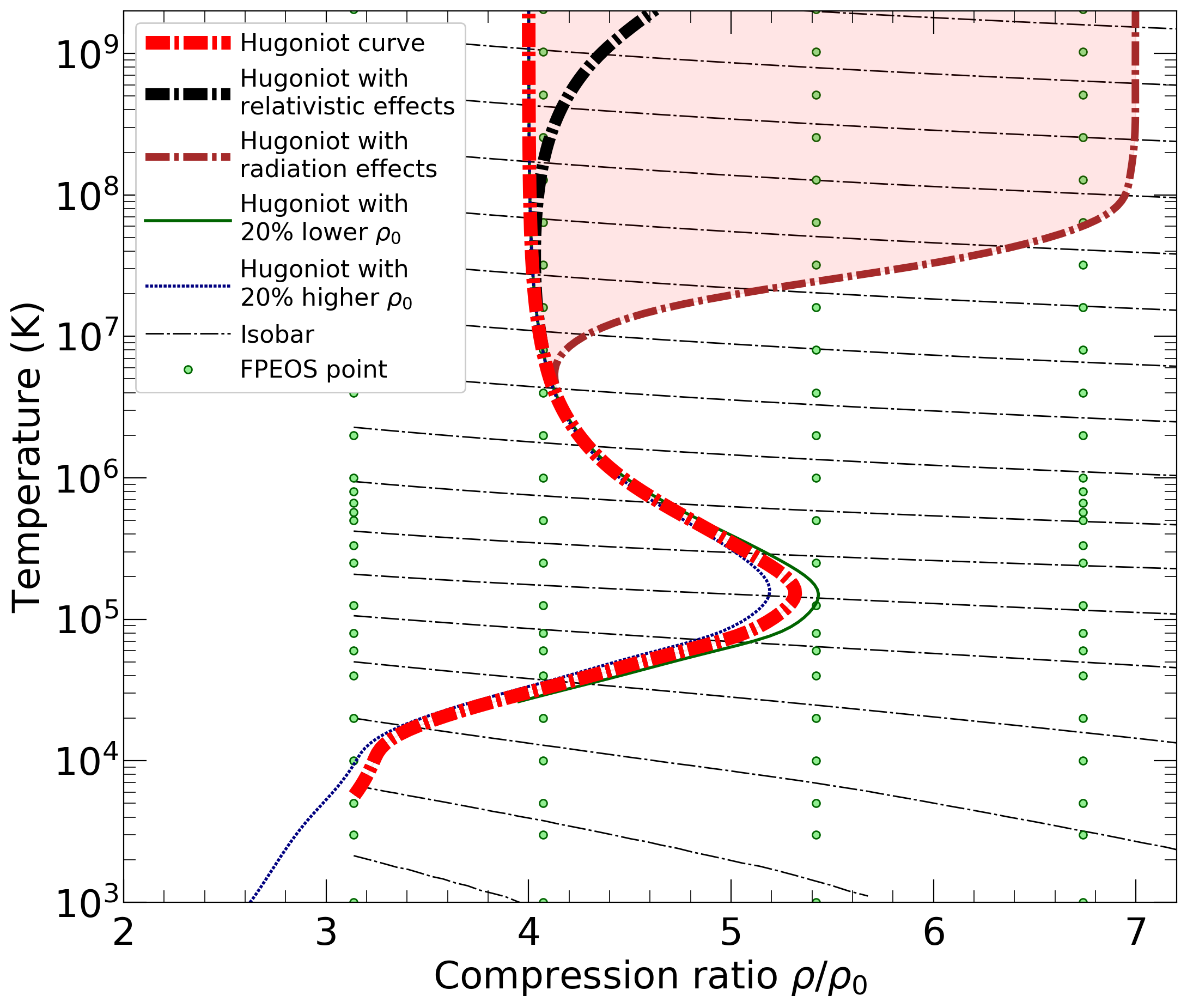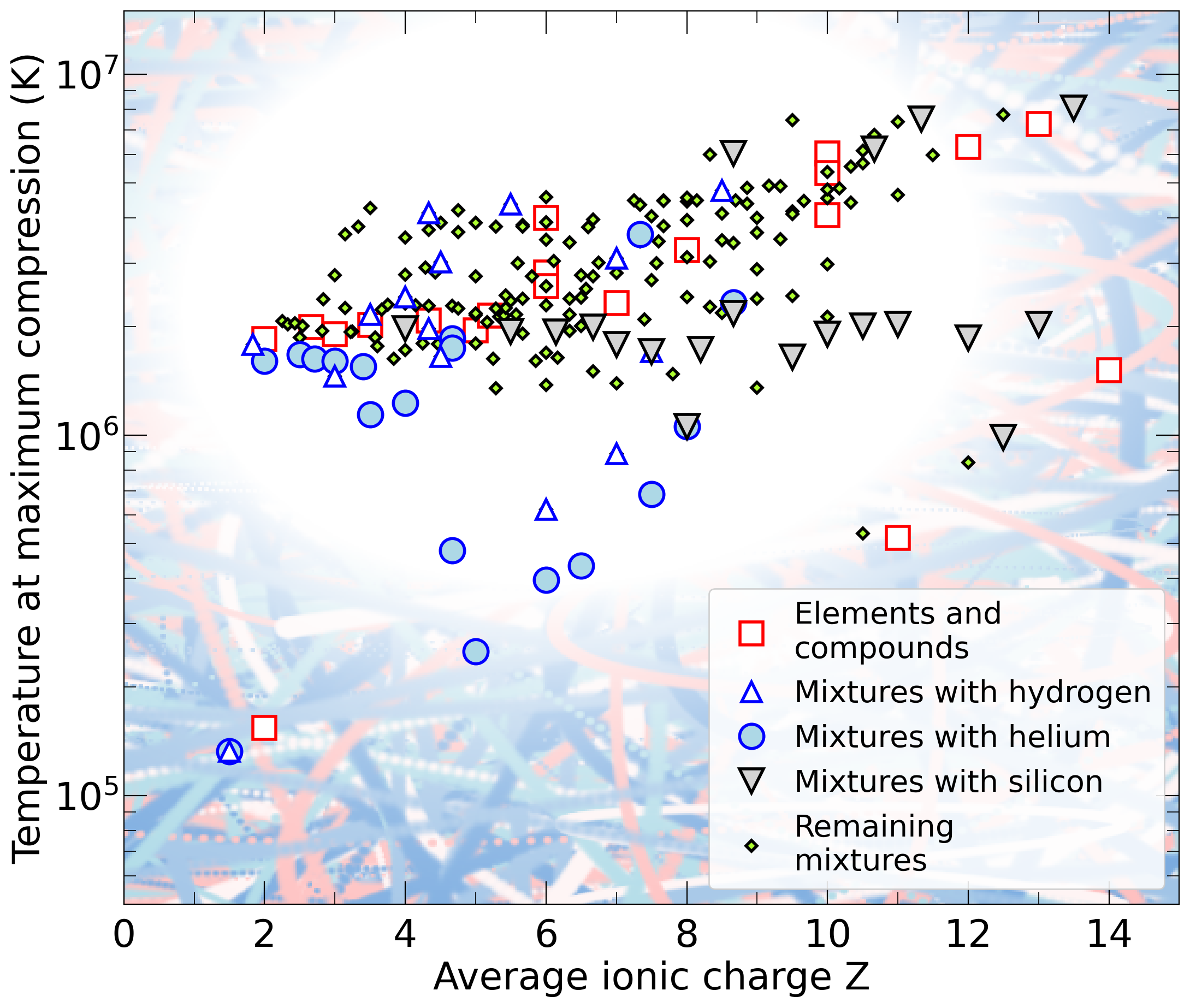(2) Installation

which will call Gnu's C++ compiler g++ with the argument -O3. The output should be

g++ -O3 -c FPEOS_29.C
g++ -O3 -c EOSTableSimple.C
g++ -O3 -c Parser.C
g++ -O3 -c Standard.C
g++ -O3 -c Form.C
g++ -O3 -c Physics.C
g++ -O3 -c Spline.C
g++ -O3 -c FermiGas.C
g++ -O3 -c RelativisticFermiGas.C
g++ -O3 -c Debye.C
g++ -O3 -c GKIntegration.C
g++ -O3 -c RungeKutta.C
g++ FPEOS_29.o EOSTableSimple.o Parser.o ReadInTable.o Standard.o Form.o Physics.o Spline.o FermiGas.o RelativisticFermiGas.o Debye.o GKIntegration.o RungeKutta.o -lm -o fpeos

In case this fails, try editing the Makefile or switch to a different compiler.
The code has been compiled successfully with these three compilers:

Linux g++ version 4.8.4 (Ubuntu 4.8.4-2ubuntu1~14.04.4)
Linux g++ version 7.5.0 (Ubuntu 7.5.0-3ubuntu1~18.04)
Apple clang version 12.0.0 (clang-1200.0.32.2)

To run our code, you need a C++ compiler. Python needs to be installed to generate the graphs (but C++ code will work without Python). Linux machines typically have the g++ compiler and Python already installed.

On Macs, we recommend installing XCode with the command line tools as well as installing Python with Anaconda (but there could be more disk space conserving options to install a C++ compiler and Python).

On Windows, install Python as well as Cygwin with g++ version 10.2.1. Then run fpeos.exe and open the resulting PDF files with cygstart.

(3) Files included

The EOS tables are stored in files

 Al_EOS_09-18-20.txt C16H24_EOS_09-18-20.txt LiF_EOS_09-18-20.txt Ne_EOS_09-18-20.txt B4C_EOS_09-18-20.txt C18H18_EOS_09-18-20.txt MgO_EOS_09-18-20.txt O_EOS_09-21-20.txt BN_EOS_09-18-20.txt C20H10_EOS_09-18-20.txt MgSiO3_EOS_09-18-20.txt Si_EOS_10-19-20.txt B_EOS_09-18-20.txt C_EOS_09-18-20.txt Mg_EOS_09-18-20.txt C10H40_EOS_09-18-20.txt H_EOS_09-18-20.txt N_EOS_09-18-20.txt C14H28_EOS_09-18-20.txt He_EOS_09-18-20.txt Na_EOS_09-18-20.txt

In the every file, you find a header followed by blocks of data, one for every density. Our carbon EOS file begins with

f= C N= 1 rho[g/cc]= 0.100006 V[A^3]= 199.43071700 T[K]=  5000 P[GPa]= 0.053 0.007 E[Ha]= -37.66843800 0.00054900
f= C N= 1 rho[g/cc]= 0.100006 V[A^3]= 199.43071700 T[K]= 10000 P[GPa]= 0.182 0.022 E[Ha]= -37.56017000 0.01215800
f= C N= 1 rho[g/cc]= 0.100006 V[A^3]= 199.43071700 T[K]= 50000 P[GPa]= 5.670 0.013 E[Ha]= -36.69107600 0.00495700

The columns provide the following information:
 2) The chemical formula, such as C, MgSiO3, C10H40, etc. This entry is not used to normalized V and E. 4) Number of atoms, N, that are used to normalized the extensive quantities V and E in later columns. 6) Density in gramm/cm3 using standard masses for all atoms. 8) Volume of N atoms in units of Angstroem3 (1 Angstroem = 10-10 meters = 1/0.529177208607 aBohr). This is directly taken from the simulations and does not depend on the assumed atomic masses. 10) Temperature in units of Kelvin (K). 12) Pressure derived from PIMC or DFT-MD simulations in units of 109 Pascals. 13) 1-sigma error bar of pressure that was derived when the ensemble average was computed. 15) Internal energy per N atoms in units of Hartrees (1 Ha = 4.359743802665602 * 10-18 J = 27.21138342791109 eV = 315774.6608728946 K * kb). The zero of energy is that of a fully ionized system so that an hydrogen atom has the energy of -0.5 Ha = -13.6 eV. The means all VASP energies need to be shifted but those from Cupid, Abinit, Quantum Espresso, and most quantum chemistry codes do not. 16) 1-sigma error bar of the internal energy that was derived when the ensemble average was computed.

The C++ files are

 Array.C Form.C Random.h Array.h Form.h ReadInTable.C DataElement.h Function.h ReadInTable.h Debye.C GKIntegration.C RelativisticCorrectionsFermiGas.h Debye.h GKIntegration.h RelativisticFermiGas.C EOSTableSimple.C HeapSort.h RelativisticFermiGas.h EOSTableSimple.h IdealMixture.h RungeKutta.C Elements.h OrderedArray.h RungeKutta.h FPEOS_29.C ParseCommandLineArguments.h Spline.C FermiGas.C Parser.C Spline.h FermiGas.h Parser.h Standard.C FindMinimum.h Physics.C Standard.h FindRoot.h Physics.h Vector.h

The C++ code calls the following Python scripts to write graphics files in .png and .pdf formats.

 FPEOS_P_T_plot03.py FPEOS_comp_P_plot04.py FPEOS_mixture_rho_T_plot01.py FPEOS_T_E_Debye_plot03.py FPEOS_comp_T_plot03.py FPEOS_mixture_up_us_plot01.py FPEOS_T_E_Ideal_plot03.py FPEOS_mixture_P_T_plot01.py FPEOS_rho_T_plot03.py FPEOS_T_P_Debye_plot03.py FPEOS_mixture_comp_P_plot01.py FPEOS_up_us_plot01.py FPEOS_T_P_Ideal_plot03.py FPEOS_mixture_comp_T_plot01.py

These files should work with Python 2.7 and 3.7 as long as the following import commands work:

from matplotlib.pylab import *
import numpy
import sys
import os

Finally there is a "readme.txt" file.

(4) Execution

To obtain the latest instructions type

fpeos

which will print the elements and compounds in the database:

 1: Hydrogen 8: Sodium 15: CH4 2: Helium 9: Magnesium 16: CH2 3: Boron 10: Aluminum 17: CH1.5 4: Carbon 11: Silicon 18: CH 5: Nitrogen 12: LiF 19: CH0.5 6: Oxygen 13: B4C 20: MgO 7: Neon 14: BN 21: MgSiO3

You can run our fpeos code in the following ways:

 For helium EOS: fpeos EOS=2 For B4C EOS: fpeos EOS=13 For MgSiO3 EOS: fpeos EOS=21 For H2+O mixture: fpeos binaryMixture EOS1=1 2.0 EOS2=6 1.0 For He+Ne mixture: fpeos binaryMixture EOS1=2 1.0 EOS2=7 1.0 For H+He mixture: fpeos binaryMixture EOS1=1 0.92 EOS2=2 0.08 For MgO+MgSiO3 mixture: fpeos binaryMixture EOS1=20 1.0 EOS2=21 1.0 For C+O mixture: fpeos binaryMixture EOS1=4 1.0 EOS2=6 1.0 rho0=1.0426 E0=-112.9115 For C+O2 mixture: fpeos binaryMixture EOS1=4 1.0 EOS2=6 2.0 rho0=1.6381 E0=-188.1576

rho0[g/cc] and E0[Ha/FU] overwrite the default values for initial conditions of Hugoniot curves.

The extra numbers are number fractions that define the formula unit (FU), which is used to normalize extensive quantities like volume and energy.
 To normalize per C2H6 unit, write: fpeos binaryMixture EOS1=15 1.0 EOS2=16 1.0 To normalize per CH3 unit, write: fpeos binaryMixture EOS1=15 0.5 EOS2=16 0.5 To normalize per atom, write: fpeos binaryMixture EOS1=15 0.125 EOS2=16 0.125

The FPEOS code will write a number of .txt files, then call Python to make plots, and finally try to open the .pdf files. To identify all new output files, type "ls -ltr"

Add the options "doNotCallPython" or "doNotOpenPDFFiles" if you prefer. The arguments "processAllEOSs", "processAllMixturesOfElements", "processAllMixtures", "hugoniotCompressionMaximum", and "noLogInterpolation" are available also.

(5) Resulting files

In standard Linux fashion, type "ls -ltr" to figure out, which files have been written.
For "fpeos EOS=13", the result should be

The .txt files were all written by the fpeos executable. The .png and .pdf files were produced by the Python scripts that fpeos called.

If you have feedback, comments, or a specific scientific problem you would like to solve please send email to militzer[at]berkeley[dot]edu.

Best wishes and happy FPEOS computation!

Burkhard Militzer
January 12, 2021.